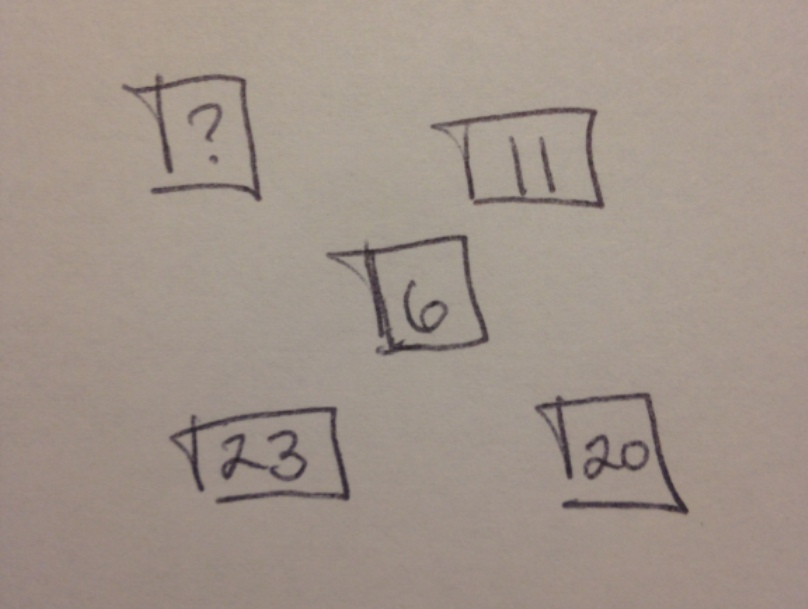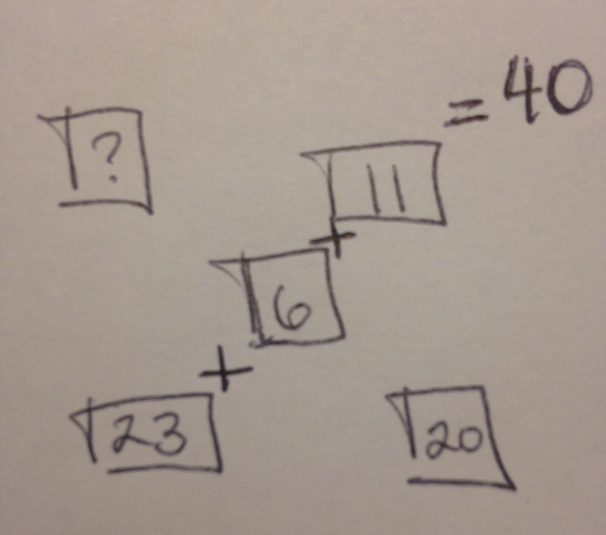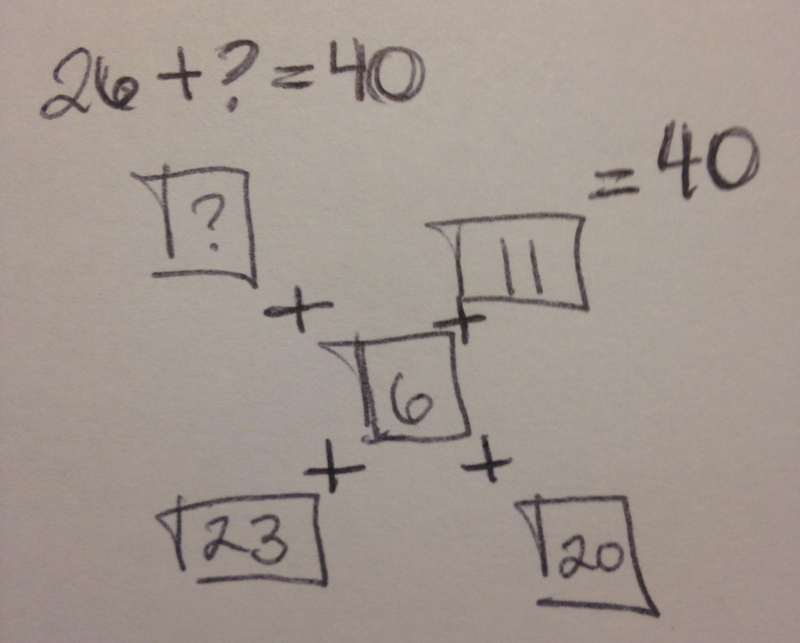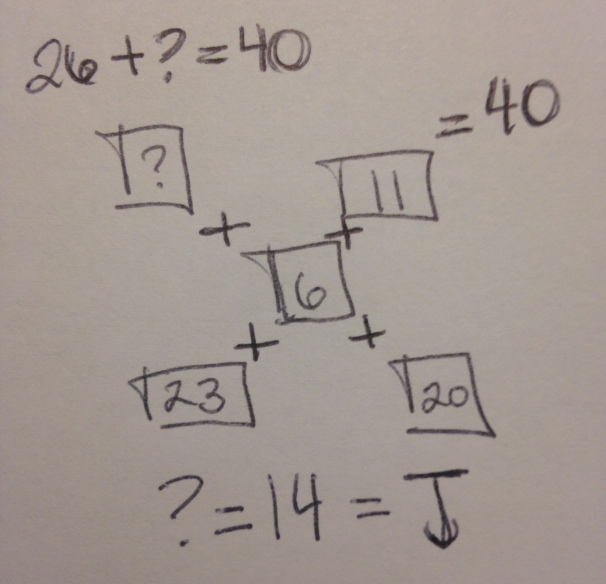•For parents, math can be confusing especially when they've been out of the school system for decades. But even we think this is a bit much for the typical first grader.

The logic puzzle, seen below, uses a key of letters to replace numbers in no discernible pattern.

So, we decided to give this a shot and "show your work" like the hashtag requested. We first replaced all letters with their representative numerical value:We then decided to add the diagonals. Assuming the homework is truly intended for younger kids, any pattern more complex than that would be straight up cruel.Adding up the diagonal with all three gave us a sum of 40. Thus (assuming our theory is correct), the alternative diagonal will also equal 40.Basic math kicks in here. 40 - 26 = 14. A quick check on the key tells us that 14 = J. Thus, J is our final answer.However, not everyone appreciated the brainteaser. One commenter said:

Well, like most math, there isn't a ton to "hone" other than, ya know, basic logic and pattern skills...

Most Popular